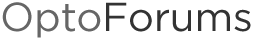# DewPoint, Enthalpy, WetBulb calculations

Hi All,

Recently we helped a customer who needed to do some Dew Point, Wet Bulb and Enthalpy calculations. It took some hunting to find all the pieces in a usable format (one we could convert to OptoScript).

I’ve attached the PAC Project Pro subroutines (let me know if you’d like me to email you the OptoScript if you have PAC Control Basic).

As an example, here’s the OptoScript to calculate Enthalpy from Dry Bulb Temperature (vs. Wet Bulb): (this is actually OptoScript, not PHP, but as you’ll see below, the logic/syntax wasn’t much different than an old BASIC example we found)

``````
-------------------------------------------------------------------------------------------------------------

Here's a short BASIC program that calculates the enthalpy using dry bulb temperature and relative humidity.

10 REM ENTHALPY CALCULATION
20 REM Assumes standard atmospheric pressure (14.7 psi), see line 110
30 REM Dry-bulb temperature in degrees F (TEMP)
40 REM Relative humidity in percentage (RH)
50 REM Enthalpy in BTU/LB OF DRY AIR (H)
60 T = TEMP + 459.67
70 N = LN( T ) : REM Natural Logrithm

80 L = -10440.4 / T - 11.29465 - 0.02702235 * T + 1.289036E-005 * T ^ 2 - 2.478068E-009 * T ^ 3 + 6.545967 * N
90 S = LN-1( L ) : REM Inverse Natural Logrithm
100 P = RH / 100 * S
110 W = 0.62198 * P / ( 14.7 - P )

120 H = 0.24 * TEMP + W * ( 1061 + 0.444 * TEMP )
*/

if (TMode_In_n) then
Tdb_degF_f = (9.0/5.0) * DryBulbT_f + 32.0;//T Mode In = 1  convert Dry Bulb temperature from Celsius to Fahrenheit
else
Tdb_degF_f = DryBulbT_f;//T Mode In = 0  Dry Bulb Temperature in Fahrenheit
endif

BASIC_T_f = Tdb_degF_f +  459.67; // deg Kelvin conversion?
BASIC_N_f = NaturalLog(BASIC_T_f); //Natural Logrithm
BASIC_L_f = (-10440.4 / BASIC_T_f) - 11.29465 - (0.02702235 * BASIC_T_f) + 0.00001289036 * Power(BASIC_T_f, 2) - (0.000000002478068 * Power(BASIC_T_f, 3)) + 6.545967 * BASIC_N_f;
BASIC_S_f = RaiseEToPower(BASIC_L_f);
BASIC_P_f = (Relative_Humidity_f / 100.0) * BASIC_S_f;
BASIC_W_f = 0.62198 * BASIC_P_f / ( 14.7 - BASIC_P_f );

BASIC_H_f = 0.24 * Tdb_degF_f + BASIC_W_f * ( 1061.0 + 0.444 * Tdb_degF_f );

``````

Of course, the usual disclaimers apply. Hope that helps!

-OptoMary

9_3Pro.zip (7.68 KB)

Also see Don’s post here for another method of getting Dew Point.